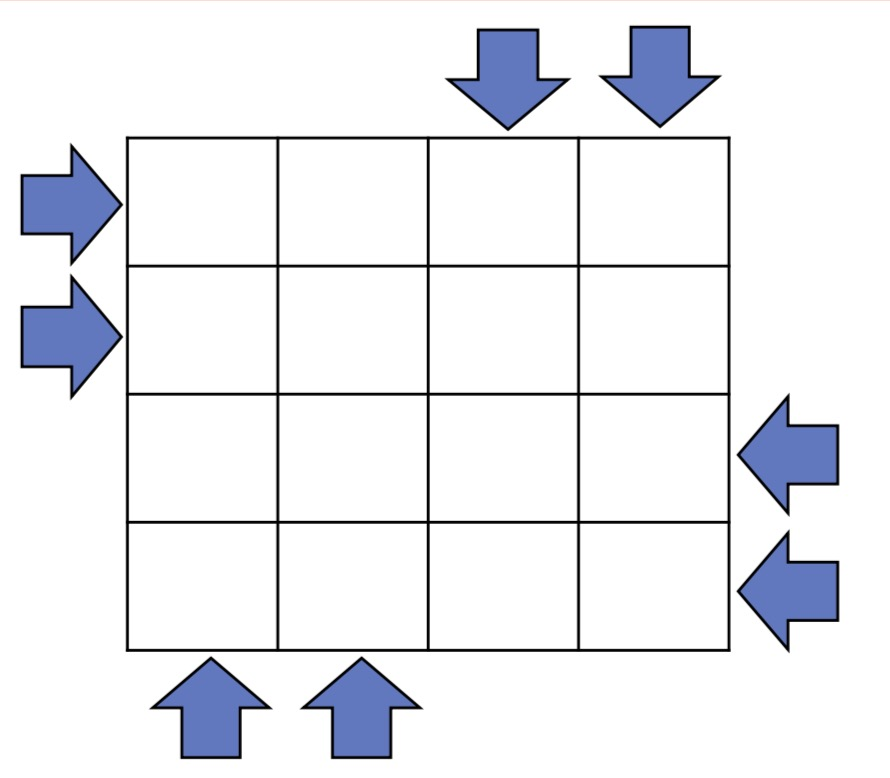# A.run

## 题解

1步2步上楼梯的加强版。典型的dp题。

• $dp[i][0/1]$表示第$i$米最终是走还是跑的方案数

• $dp[i] = dp[i-1]+dp[i-1]$
• $dp[i] = dp[i-1]$

## D.Money

### 题解

dp

$dp[i]$表示在$i$最后一次交易是买入的最优值，$dp[i]$表示在$i$最后一次交易是卖出的最优值。转移方程。

• 在$[1,i-1]$中卖出的最优值后在$i$买入或者不买入中取最好值。$dp[i]=max(dp[i-1],dp[i-1]-a[i])$
• 在$[1,i-1]$买入的最优值后在$i$卖出或者不卖中取最大值。$dp[i] = max(dp[i-1],dp[i-1]+a[i])$

## G transform

### 题解

• 那么如果我们将产品$[l,r]$运送到点$l$的消耗相当于把$[l,r]$中的元素都先运送到$l$中之后再把$l$中的元素送到0，进行减一哈，就是答案。$ans = sumt[r]-sumt[l-1]-(suma[r]-suma[l-1])*x[l]$
• 将产品$[l,r]$运送到$r$点，相当于我们先把$[l,r]$转移到$r$上面之后再统一移动到0点。$ans+sumt[r]-sumr[l-1]=(suma[r]-suma[l-1])*x[r]$。

### AC代码

https://www.nowcoder.com/discuss/88268?type=101&order=1&pos=4&page=1

https://www.cnblogs.com/Flower-Z/p/9528057.html

## H.travel

### 题解

$f[i][j]$表示以$i$为根的子树选了$j$条路径的最大权值和。(也是包含$i$点)

$g[i][j]$表示以$i$为根的子树选了$j$条路径加上一条包含$i$到根节点链的最大权值。

• $g[u][i] = max(g[u][i],f[v][x]+g[u][i-x])$
• $g[u][i] = max(g[u][i],g[v][x]+f[v][i-x])$

• $f[u][i]=max(f[u][i],f[v][x]+f[u][i-x])$

## I.Car

• 速度一样
• 不能改变方向
• 起点都在矩阵的边缘

### 题解## J.Farm

### 题解

$a$植物代号是101010,那么检查每一位的时候，101011肯定对它不好，101110在检查前几位的时候可能对它好。

• 他加入对它好的次数是不是总的施在他身上的次数。如果不等，说明有其他不好的施肥了，那他就挂了。
• 他加入对它不好的次数存不存在，如果有，那他也挂了。